telescopeѲptics.net          ▪▪▪▪                                             CONTENTS

# 10.2.2.4. Schmidt-Cassegrain telescope (SCT)

PAGE HIGHLIGHTS
Spherical aberration   • Spherochromatism

Among the most popular commercial designs, the Schmidt-Cassegrain telescope (or SCT, FIG. 174) owes its success mostly to the possibility of relatively inexpensive commercial production of a well-performing Schmidt corrector, particularly in combination with two spherical mirrors. The road for the SCT commercial production was paved by Celestron's Tom Johnson who, back in the '60-ties, pioneered the method for corrector's mass-production.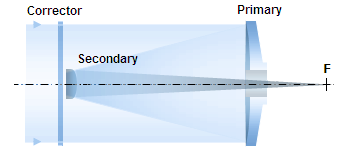FIGURE 174: Schmidt-Cassegrain telescope is a Cassegrain-like two-mirror system combined with a full-aperture Schmidt corrector. Various combinations of corrector separation and mirror conics are possible, with somewhat different image field properties. Prevailing commercial arrangement is a compact design with fast spherical primary and usually also spherical secondary mirror, resulting in ~ƒ/10 system. All-spherical SCT is corrected only for spherical aberration, with low astigmatism, as well as relatively strong field curvature and coma remaining. The corrector also induces low level spherochromatism, undetectable visually and negligible for most photographic applications.

Optical effect of the corrector on the system parameters is small, but not negligible. It slightly increases the marginal ray height on the primary, as determined by its refraction angle δ (Eq. 107), with this ray being then directed toward a different focus focus point, as a result of corrector's interference.

With, say, 200mm ƒ/2 spherical primary, combined with a 0.707 neutral zone corrector at σ1=0.4R1 (primary's radius of curvature) in front of it, marginal ray on the primary will be only ~0.23mm higher (for ~0.71 corrector power in an all-spherical arrangement). The two, corrector and primary, "act" as a prolate ellipsoid (K~-0.71), nearly 200.5 mm in diameter, with only slightly extended marginal focus. Since it still retains ~29% of the original D(mm)/32F longitudinal spherical aberration, in order to find out secondary magnification we need to trace the 0.707 zone ray, the only one whose height and orientation after passing the corrector and primary didn't change, and to whose focus the rest of rays will be directed after reflection from the secondary.

Taking the 0.707 ray as marginal, the primary becomes 141.4mm diameter ƒ/2.79 mirror (the 0.707 ray focuses at the mid point of the original longitudinal defocus, 1.56mm inside the primary's paraxial focus). Slightly shorter focal length - and the corresponding radius of curvature - increase the effective secondary-to-primary radius of curvature ratio ρ from 0.3125 (in an ƒ/2/10 mirrors-only system with paraboloidal primary) to 0.3137, with the relative ray height at the secondary in units of the aperture radius k reduced from 0.25 to 0.2471, and the resulting secondary magnification m reduced to ~4.7.

Applying this magnification value to the effective 200mm ƒ/1.992 primary results in a final ƒ/9.38 system. So, if the two mirrors without corrector would form an ƒ/10 system, optical effect of the corrector changes it into ~ƒ/9.4. In order to have an ƒ/10 system with an ƒ/2 primary, the secondary needs to be slightly more (~1.5%) strongly curved, thus with the R2/R1 radii ratio ρ~0.308, for the secondary magnification m=~5.05. The relative back focal distance in units of the primary focal length is only slightly reduced, from 0.5 to η~0.49.

Aberration-wise, there are two significant differences between the SCT and all-reflecting Cassegrain varieties. One is that the SCT can be made free from both, coma and astigmatism, while an all-reflecting arrangement can only correct for one. On the other hand, the Schmidt corrector induces some sphero-chromatism. Follows overview of SCT axial aberrations, spherical (as a sum of spherical aberration contributions of its three elements) and spherochromatism.

SCT spherical aberration

The only significant monochromatic aberration introduced by the Schmidt corrector in collimated light is spherical. Its purpose is to offset spherical aberration on the two mirrors, resulting in a spherical-aberration-free system. Thus, the P-V wavefront errors for lower-order spherical aberration at the best focus for an SCT system can be written as:with scr being the corrector spherical aberration coefficient, d the pupil (aperture) radius and the mirror aberration coefficient sM being the sum of the individual mirror spherical aberration coefficients, sM=s1+s2. The individual mirror coefficients for object at infinity are same as for a two-mirror system alone (Eq. 9.2 and Eq. 9 for the primary and secondary, respectively), given by: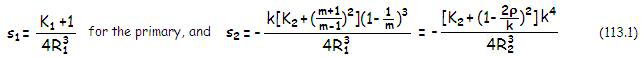for the secondary, with K1 being the primary conic, R1 the primary radius of curvature, k the height of marginal ray at the secondary in units of the aperture radius, m the secondary magnification and ρ=R2/R1, the secondary radius of curvature in units of R1.

Note that in order to be able to directly add the two coefficients, the secondary aberration coefficient had to be corrected for the difference in apertures by multiplying it with k4 factor, the relative height of marginal ray at the secondary in units of the ray height at the primary. The two forms for the secondary aberration coefficient have parameters interchangeable through k/ρ=kR1/R2=(m-1)/m=1-(1/m).

Thus, the system P-V wavefront error at the best focus can be written as: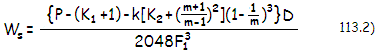with P being the needed corrector power to cancel system spherical aberration, and ss={}/4R13 being the system aberration coefficient.

Of course, for zero system spherical aberration, the aberration coefficient for the corrector scr, which is related to the corrector power P as scr=-P/4R13, needs to be equal to the sum of mirror aberration coefficients sM, and of the opposite sign. If the primary is spherical, to cancel its aberration alone, the corrector aberration coefficient needs to be scr=-b/8 (with the aspheric term b=2/R13, it comes to scr=-1/4R13, same as the spherical primary, but of the opposite sign). The corrector with this value of the aspheric term is said to have a unit power P. In such arrangement, for cancelled spherical aberration of the system, the secondary mirror conic needs to be K2=-[(m+1)/(m-1)]2, same as in the classical Cassegrain. With both, primary and secondary spherical (K1=K2=0), needed power P of the corrector for zero system spherical aberration is (from Eq. 113.1) P=1-k(m-1)(m+1)2/m3. For the typical ƒ/2/10 SCT with k~0.25 and m~5, P~0.71, i.e. needed corrector strength, or depth, is about 71% of that needed to correct the primary alone. In terms of the F-ratio, this corrector has the strength needed to correct some 12% slower primary.

In general, for any combination of conics, needed corrector power to cancel spherical aberration of two mirrors is determined by a value of the sum of opposite aberrations contributions of the primary and secondary relative to spherical aberration of the primary alone. Thus, it can be written as P=1-(s'2/s'1), with the aberration contribution of the secondary s'2 in proportion to k[K2+(m+1)2/(m-1)2](1-1/m)3, and aberration contribution of the primary s'1 proportional to (K1+1). The prime notation is to differentiate the proportionate relative contributions from the corresponding actual aberration coefficients s1=s'1/4R13 and s2=s'2k4/4R23.

Thus, the lower-order aspheric parameter A1 for the corrector in a Schmidt-Cassegrain system can be, analogously to the Schmidt camera, written as A1=b/8(n'-n), but with the corresponding aspheric coefficient b changed in proportion to the needed corrector's power, as b=2P/R13. Reduction of the 5th order aspheric parameter of the SCT corrector, A2, relative to the value for primary alone, is typically greater than that of A1, due to significant higher-order spherical aberration of opposite sign generated at the secondary as a result of relatively close object (i.e. image of the primary) distance, as well as due to reduction in the higher-order aberration resulting from reduced corrector separation (i.e. height of marginal ray at mirror surface). For spherical secondary and typical 8" ƒ/2/10 SCT configuration with σ~0.4, the parameter, given by A2=b'/16(n'-n), with the higher-order aspheric coefficient b' approximately 1/6 of that needed for primary alone (with the stop at the center of curvature), or b'~[1-(k6/ρ5)]/R15.

Thus, the higher-order corrector's power in the typical commercial unit is only ~0.16 of that needed to correct higher-order spherical aberration of a comparable ƒ/2 Schmidt system. The parameter changes for different values of σ1 approximately in proportion to σ1/0.4, thus the generalized approximation for b' can be written as b'~2.5σ1[1-(k6/ρ5)]/R15.

For closer objects, spherical aberration coefficients for all three, corrector, primary and secondary change (it is negligible for the corrector), disturbing presumed  near-zero balance for distant objects, and resulting in spherical aberration. The chage of aberration contribution on the two SCT mirrors is similar to that in all reflecting two-mirror systems (Eq. 92). Main difference is with SCT systems that focus by moving the primary. Here, the error induced by a relatively small object distance is in part offset by under-correction induced by refocusing, which requires an increase in mirror separation.

More specifically, reduced object distance lowers under-correction of the primary, and increases over-correction of the secondary. That makes the system over-corrected; the increased mirror separation needed to bring the focus point to its fixed location diminishes the effective diameter of the secondary, reducing over-correction induced by it, and by that the overall system over-correction as well. This makes a typical commercial SCT better suited for terrestrial observations than a similar system with fixed-mirror focusing. More details on this subject are given in 11.5.2. SCT focusing errors.

SCT spherochromatism

Spherochromatism in the SCT originates at the corrector, whose corrective power is optimized for one - usually green/yellow - wavelength. Since shorter wavelengths refract more strongly, and the longer ones refract more weakly, the effective corrector power increases toward the former, and decreases toward the later. With the combined spherical aberration of the two mirrors being undercorrection, this means that shorter wavelengths (blue/violet) will be overcorrected, and the longer ones (red) undercorrected. This wavelength-dependant spherical aberration increasing with the refractive index differential vs. optimized wavelength is the only source of chromatism in a SCT.

Given aperture and F#, SCT spherochromatism is proportional to the relative power of the Schmidt corrector P. In other words, to the relative value of the aspheric term b needed to cancel spherical aberration of the system. It can be written as: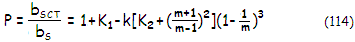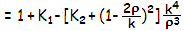with bs being the aspheric factor - or the corrector's spherical aberration coefficient - needed to correct for spherical aberration of the primary alone. As mentioned, b=2/R13, from the general form of the aspheric coefficient b=2n[(m+1)/(m-1)]2/R13, for mirror magnification m=0 (object at infinity) and index of incidence n=1.  Aspheric coefficient cancelling the aberration of a spherical mirror is bs=-b/8=-1/4R13, while  that for an SCT system,  equal to its aberration coefficient scr, is bSCT=-P/4R13, where P is the corrector power, positive in sign.

The P-V wavefront error of spherochromatism for a particular SCT arrangement is obtained by multiplying relative power of its corrector (Eq.  118) with the wavefront error for the unit power corrector (Eq. 106). For the transverse aberration (ray spot diameter), the relative power is to be multiplied with the transverse aberration for the unit power corrector (Eq. 107.1) and the SCT secondary mirror magnification.

In general, SCT spherochromatism is low. For a typical commercial ~ƒ/2/10 version, with both mirrors spherical, k~0.25, σ~0.4 and m~5, relative corrector power ~0.72, and 0.866 neutral zone placement, the red (C-line) and blue (F-line) geometric blurs are still within the Airy disc. For the 0.707 radius neutral zone placement (FIG. 175), the blurs are doubled, but the wavefront error halves for the lowest chromatism level achievable with the Schmidt corrector.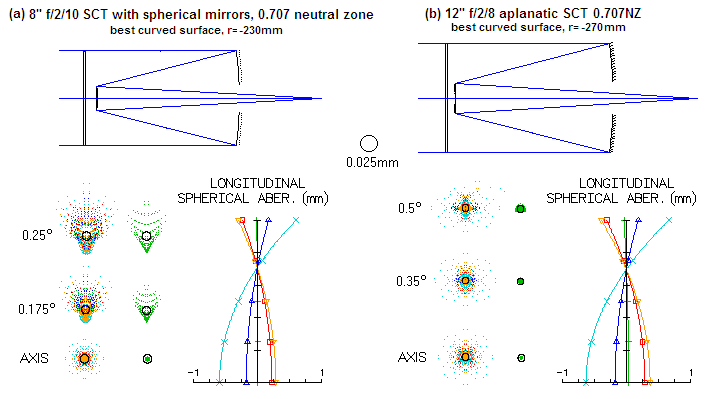FIGURE 175
: Ray spot plot of the typical commercial 8" SCT with spherical mirrors (a) and the more recent aplanatic variety (b). The 0.5° field radius is at the limit of the usable field (due to vignetting by the baffle tube), visible only in 30mm to 50mm f.l. eyepieces. Thus, the ~1/20 mm sagittal coma of the 8" is 5-6 arc minutes in the eyepiece, which is acceptable for the field edge. Its color error is only significant in deep violet: the blur size of 5-6 Airy disc diameters at the best focus location corresponds to ~0.12 wave RMS of spherical aberration. The red and blue blurs are approximately 1.5 times the Airy disc diameter, or ~0.04 wave RMS level. A 4"
ƒ/12 doublet achromat has blue/red blurs ~3.2 times the Airy disc diameter, but the error is defocus, thus worth ~0.3 wave RMS. The SCT RMS error is roughly 10 times lower, making its visual chromatism all but invisible. The 12" (aplanatic SCT) has much smaller off-axis blurs due to the absence of coma and more symmetrical (astigmatic) images with nearly four times smaller wavefront error 0.5° off-axis  (0.17 vs. over 0.6 wave RMS). Most of the increase in chromatism (~40%) is due to its larger aperture (it is roughly at the level of a 4" ƒ/70 achromat).            SPEC'S

Residual spherical aberration in the system will alter spherochromatic error due to the increased error in the optimized wavelength (FIG. 176).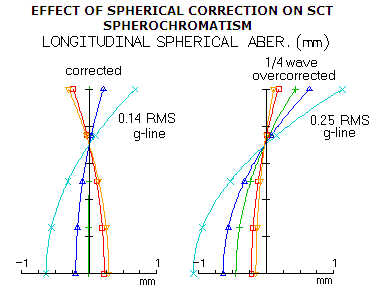FIGURE 176: Residual spherical aberration in the optimized wavelength of an SCT affects correction in all other wavelengths as well. Non-optimized wavelengths with spherical aberration at near-perfect correction of identical sign to the
e-line residual will have the aberration increased by similar amount (scaled according to the wavelength), while those with the base spherical aberration of opposite sign will have it reduced by nearly as much (generally, the error will be somewhat greater for shorter wavelength, and somewhat smaller for the longer ones).

The overall correction level is nearly certain to be worse, not only due to the error in the optimized wavelength, to which (and those relatively close to it ) the eye is most sensitive.  As the plot at right shows, the wavelengths with initially the opposite error sign (red), may only switch the sign, without major error decrease. This particular SCT would show noticeably more color in the blue/violet than when optimally corrected.

Since an SCT near-perfectly corrected for spherical aberration have blue wavelengths overcorrected, and red wavelengths undercorrected, a residual of, say, 1/4 wave P-V wavefront error of overcorrection in the optimized wavelength will increase spherochromatic error in the blue, and reduce it in the red (the plots are for a typical ƒ/2/10 8-inch SCT with spherical mirrors). If the residual spherical is relatively significant, spherical correction in the red is likely to be actually better than in the optimized wavelength.

Consequently, the instrument will have more or less pronounced chromatic imbalance, with the negatively affected portion of the spectrum (blue/violet wavelengths in this example) possibly showing noticeably inferior chromatic correction in both, visual observing and imaging.

Follows an overview of the SCT off-axis aberrations, after a quick look at the properties of the standard SCT with an off axis mask.

EFFECT OF OFF AXIS MASK ON 11-INCH STANDARD SCT

Off axis mask can be used with large-aperture SCTs in conditions of strong atmospheric turbulence, in order to significantly reduce its negative effect on image quality. The obvious negative is the reduction in aperture, which is assumed to be at least partly compensated for by significantly improved wavefront quality. There are, however, some additional, relatively minor negatives.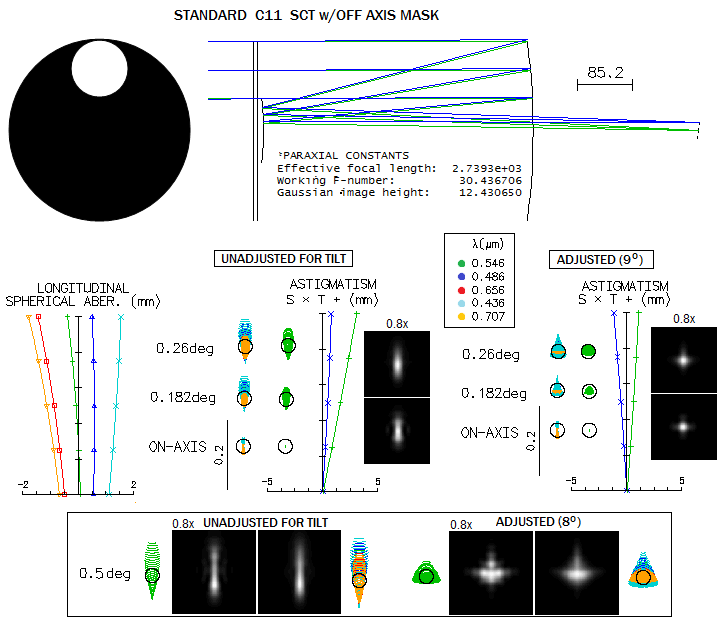Since the mask allows light to use only the upper portion of the optical train, colors are unwrapped and widely separated. Due to the very slow focal ratio, it is of little consequence if observing plane nearly coincides with the best image surface. The problem is, this surface is tilted, in this case by 9 degrees, which in the image plane perpendicular to optical axis (i.e. for unaccommodated eye) causes not only lateral deformation in the optimized color, but also lateral chromatic dispersion.

Bottom spots and images show this effect at the edge of the widest field possible with a 2-inch eyepiece barrel (best image surface tilt is slightly smaller, at 8 degrees).